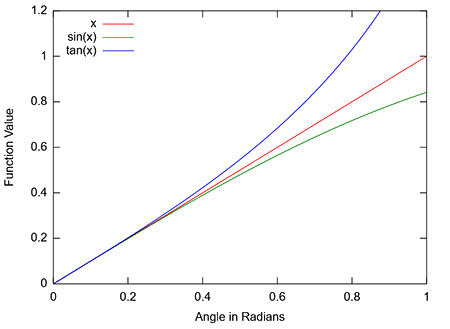# The equation for wavelength from a double-slit experiment uses the simplification that theta is...

## Question:

The equation for wavelength from a double-slit experiment uses the simplification that {eq}\theta {/eq} is small so that sin {eq}\theta {/eq} = tan {eq}\theta {/eq}.

Up to what angle is this a good approximation when your data has two significant figures? Explain.

## Trigonometry

Trigonometry is a branch of mathematics that deals with the study of angles and triangles generating relationships between each of them. In general terms, trigonometric ratios are sine, cosine, tangent, cotangent, secant, and cosecant.

The following graph shows the comparison between the values of {eq}\rm \theta, sin\theta \text{ and } tan\theta {/eq}The angle will exceed 1% error for the following angles:

{eq}\rm \tan\theta \approx \theta \rightarrow 0.1730\ rad =9.91^{\circ} \\ \sin\theta \approx \theta \rightarrow 0.2441\ rad = 13.99^{\circ} {/eq}